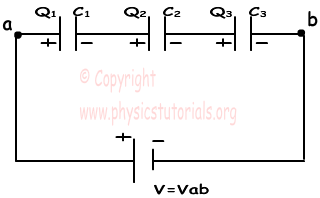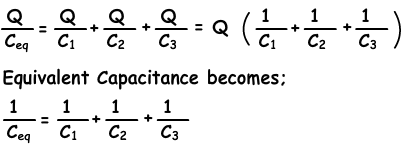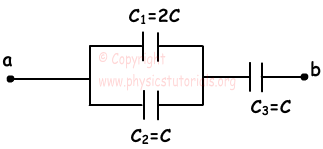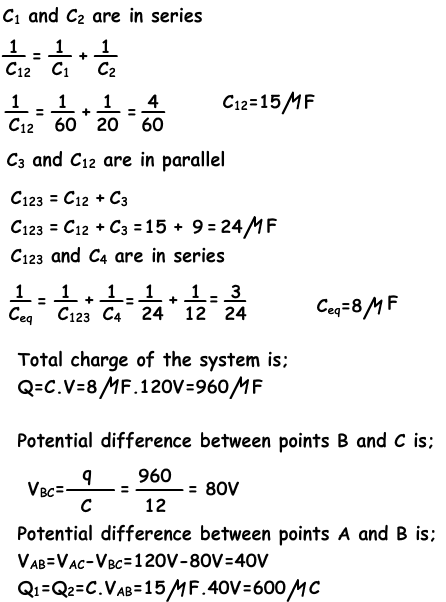• ×
السبت 6 يونيو 2020 | 05-28-2020
×

# المكثف Capacitor

Capacitors are widely used in electronic circuits. Capacitors have vast applications. They are used in almost every Machine that is working with some electronics components.
Now before reading or learning more about capacitors, we should be familiar with capacitance. You may heard this ago if you are a student of Electronics.
Capacitance:
We all know that capacitors are used to store charge. Capacitance of a capacitor is the ability of a capacitor to store the charge.

Capacitance may be defined as the amount of charge required to cause unit potential difference in a conductor.

The unit of capacitance is Farad.
One Farad is defined as the capacitance, where a one coulomb charge causes potential difference of one volt across the plates of capacitor.
Mathematically:
C = Q/V
Q = Charge (Coulomb)
V = Potential Difference (Volts)
Construction of a Capacitor:

A capacitor consists of two plates which are separated by a die electric or insulating material. When we connect a voltage source to conductor, it will  store charge.
Charge is stored in the form of Electrostatic field in a capacitor

The symbol for an Capacitor is shown as:

Working:
When voltage is applied to capacitor, there is no excess or loss of electrons on any plate of capacitor. So there is no potential difference between them. Positive Charges towards one plate and thus make that plate positive and in same way negative charges flow towards other plate and thus make that plate negative.

Now negative charges are repelled by positive plate of capacitor and in same way negative plate repells positive charges(As we know that like charges always repel each other while unlike charges always attract . each other.)Thus both charges goto the area where they in excess. This causes a potential difference between two plates. This repulsion or movement of charges will continue till the potential difference between two plates reach the value as voltage source.
Now a maximum value of current will flow and it falls to zero when voltage reaches maximum value.

# Capacitors in Series and Parallel with Examples

Capacitors in Series and Parallel

Systems including capacitors more than one has equivalent capacitance. Capacitors can be connected to each other in two ways. They can be connected in series and in parallel. We will see capacitors in parallel first.

In this circuit capacitors are connected in parallel.Because, left hand sides of the capacitors are connected to the potential a, and right hand sides of the capacitors are connected to the potential b. In other words we can say that each capacitor has same potential difference. We find the charge of each capacitor as;

Q1=C1.V

Q2=C2.V

Q3=C3.V

Total charge of the system is found by adding up each charge.

Qtotal=Ceq.V

Qtotal= Q1+Q2+Q3=C1.V+C2.V+C3.V=V.(C1+C2+C3)=Ceq

Ceq=C1+C2+C3

As you can see, we found the equivalent capacitance of the system as C1+C2+C3

Now we will see the capacitors in series;In capacitors in series, each capacitor has same charge flow from battery. In this circuit, +Q charge flows from the positive part of the battery to the left plate of the first capacitor and it attracts –Q charge on the right plate, with the same idea, -Q charge flows from the battery to the right plate of the third capacitor and it attracts +Q on the left plate. Other capacitors are also charged with same way. To sum up we can say that each capacitor has same charge with batter.

C1.V1=Q

C2.V2=Q , V=V1+V2+Vand Q=Ceq.V

C3.V3=QExample: Calculate the equivalent capacitance between the points a and b.Example: In the circuit given below, C1=60µF, C2=20 µF, C3=9 µF and C4=12 µF. If the potential difference between points a an b Vab= 120V find the charge of the second capacitor.المصدر

بواسطة :
06-01-2016 12:00 صباحاً  0  0  2.9K
التعليقات ( 0 )